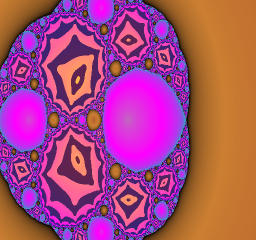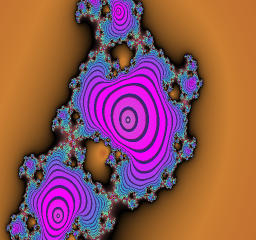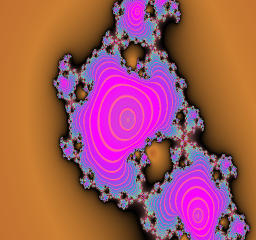## Movies of Julia sets for Blaschke Product of degree 3

Blaschke's product map is given by the following rational function of degree three.
f(z) = z^2(z+a)/(1+bz)
Where b is the complex conjugate of parameter a. In the movies of this page, parameter a is varied and the dynamic plane is colored according to the behavior of the points in the z plane.
Attractive basins of periodic attractors are color coded by the number of iterations befor the initial point falls in a small neighborhood of the attractor. The points in the Herman ring or its inverse images are colored according to the distance of the orbit from the unit circle.

## Attention! Movie files are heavy.[5MB/clip]

The module of a is 4.5 and fixed, while the argument of a is varied from 0 to pi.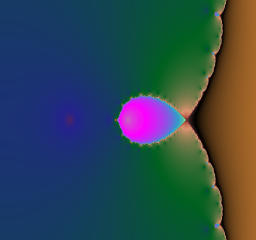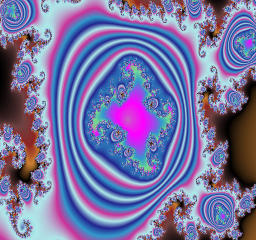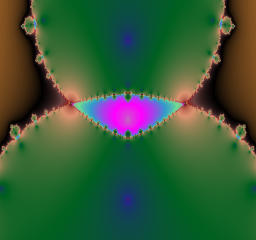The module of a is 4.1 and fixed, while the argument of a is varied from 0 to pi.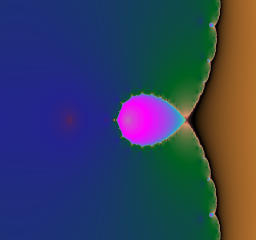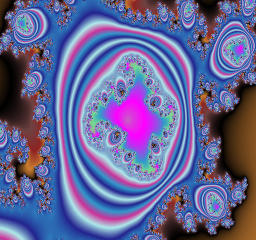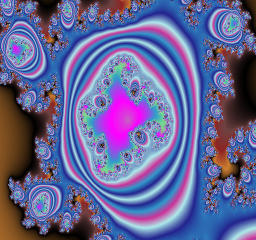The module of a is 4.0 and fixed, while the argument of a is varied from 0 to pi.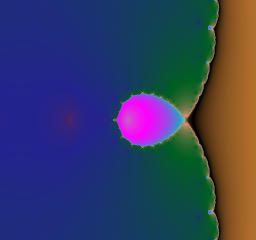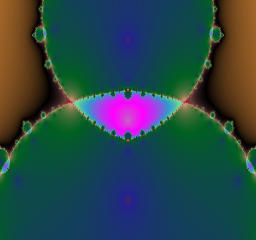The module of a is 3.5 and fixed, while the argument of a is varied from 0 to pi.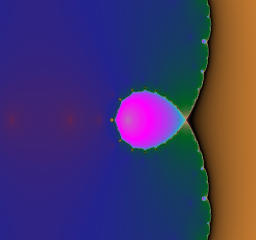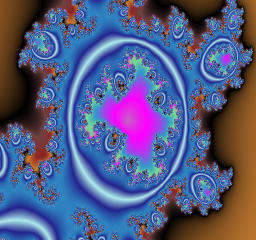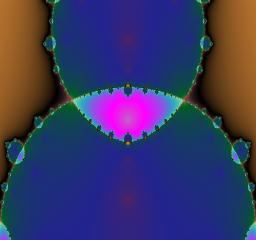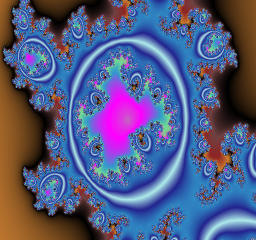The module of a is 3.3 and fixed, while the argument of a is varied from 0 to pi.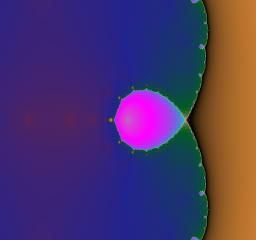The module of a is 3.2 and fixed, while the argument of a is varied from 0 to pi.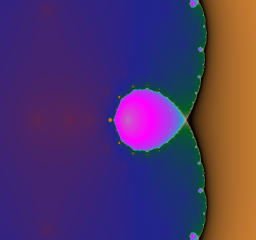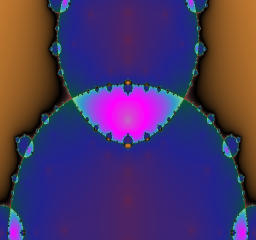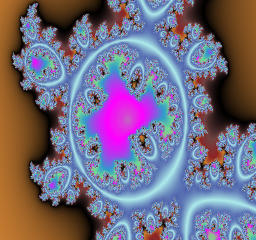The module of a is 3.1 and fixed, while the argument of a is varied from 0 to pi.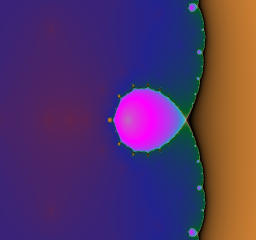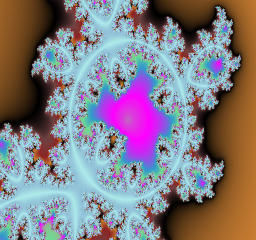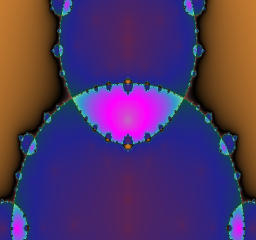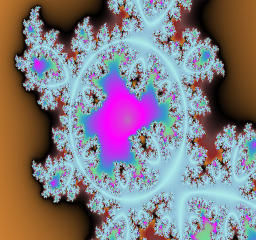The module of a is 3.0 and fixed, while the argument of a is varied from 0 to pi.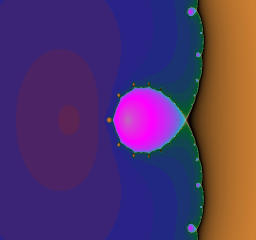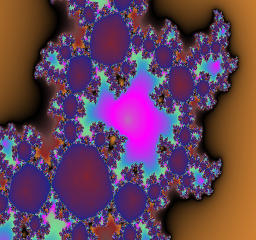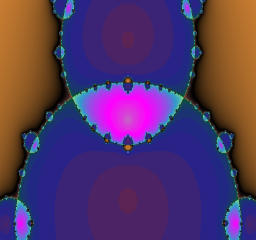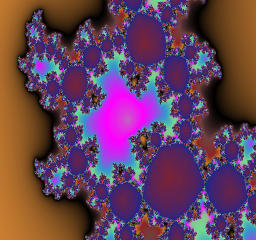The module of a is 2.9 and fixed, while the argument of a is varied from 0 to pi.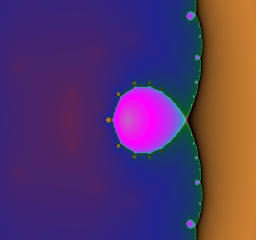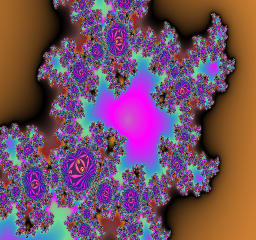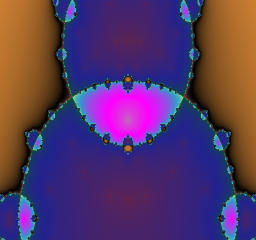The module of a is 2.5 and fixed, while the argument of a is varied from 0 to pi.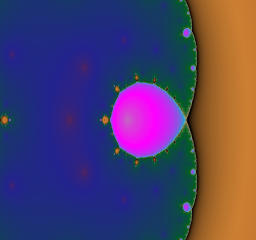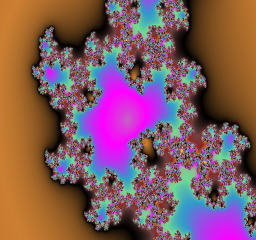The module of a is 2.1 and fixed, while the argument of a is varied from 0 to pi.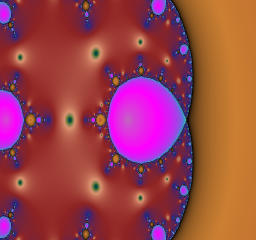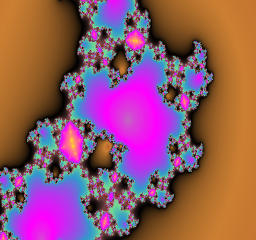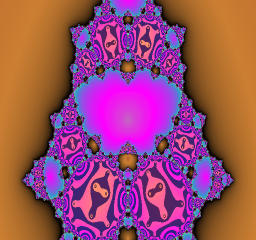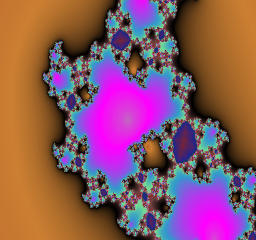The module of a is 2.0 and fixed, while the argument of a is varied from 0 to pi.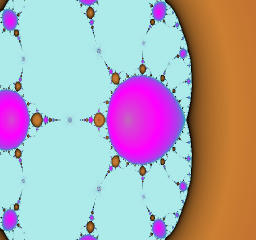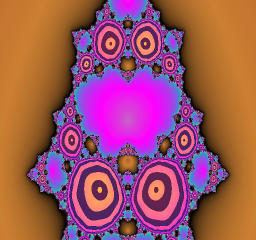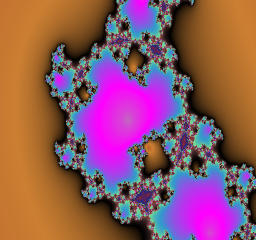The module of a is 1.9 and fixed, while the argument of a is varied from 0 to pi.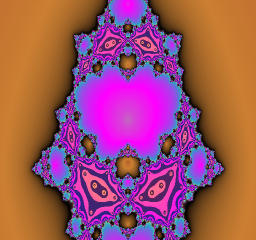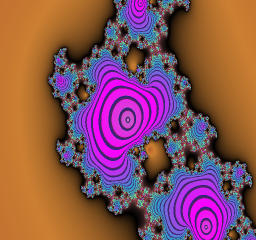The module of a is 1.7 and fixed, while the argument of a is varied from 0 to pi.